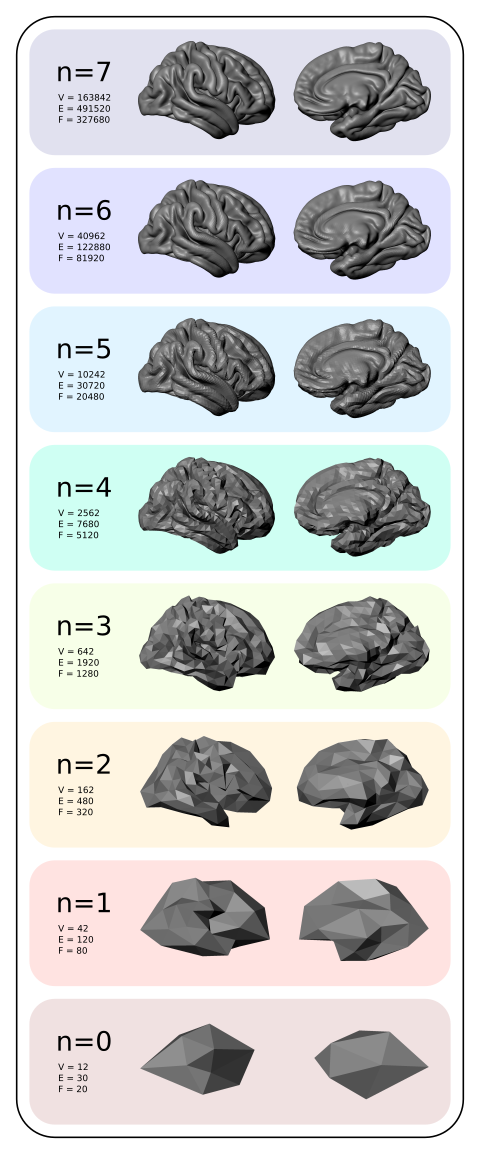# Downsampling (decimating) a brain surfaceDownsampled average cortical surfaces at different iterations (n), with the respective number of vertices (V), edges (E) and faces (F).

In the previous post, a method to display brain surfaces interactively in PDF documents was presented. While the method is already much more efficient than it was when it first appeared some years ago, the display of highly resolved meshes can be computationally intensive, and may make even the most enthusiastic readers give up even opening the file.

If the data being shown has low spatial frequency, an alternative way to display, which preserves generally the amount of information, is to decimate the mesh, downsampling it to a lower resolution. Although in theory this can be done in the native (subject-level) geometry through retessellation (i.e., interpolation of coordinates), the interest in downsampling usually is at the group level, in which case the subjects have all been interpolated to a common grid, which in general is a geodesic sphere produced by subdividing recursively an icosahedron (see this earlier post). If, at each iteration, the vertices and faces are added in a certain order (such as in FreeSurfer‘s fsaverage or in the one generated with the platonic command), downsampling is relatively straightforward, whatever is the type of data.

## Vertexwise data

For vertexwise data, downsampling can be based on the fact that vertices are added (appended) in a certain order as the icosahedron is constructed:

• Vertices 1-12 correspond to n = 0, i.e., no subdivision, or ico0.
• Vertices 13-42 correspond to the vertices that, once added to the ico0, make it ico1 (first iteration of subdivision, n = 1).
• Vertices 43-162 correspond to the vertices that, once added to ico1, make it ico2 (second iteration, n = 2).
• Vertices 163-642, likewise, make ico3.
• Vertices 643-2562 make ico4.
• Vertices 2563-10242 make ico5.
• Vertices 10243-40962 make ico6, etc.

Thus, if the data is vertexwise (also known as curvature, such as cortical thickness or curvature indices proper), the above information is sufficient to downsample the data: to reduce down to an ico3, for instance, all what one needs to do is to pick the vertices 1 through 642, ignoring 643 onwards.

## Facewise data

Data stored at each face (triangle) generally correspond to areal quantities, that require mass conservation. For both fsaverage and platonic icosahedrons, the faces are added in a particular order such that, at each iteration of the subdivision, a given face index is replaced in situ for four other faces: one can simply collapse (via sum or average) the data of every four faces into a new one.

## Surface geometry

If the objective is to decimate the surface geometry, i.e., the mesh itself, as opposed to quantities assigned to vertices or faces, one can use similar steps:

1. Select the vertices from the first up to the last vertex of the icosahedron in the level needed.
2. Iteratively downsample the face indices by selecting first those that are formed by three vertices that were appended for the current iteration, then for those that have two vertices appended in the current iteration, then connecting the remaining three vertices to form a new, larger face.

## Applications

Using downsampled data is useful not only to display meshes in PDF documents, but also, some analyses may not require a high resolution as the default mesh (ico7), particularly for processes that vary smoothly across the cortex, such as cortical thickness. Using a lower resolution mesh can be just as informative, while operating at a fraction of the computational cost.

## A script

A script that does the tasks above using Matlab/Octave is here: icodown.m. It is also available as part of the areal package described here, which also satisfies all its dependencies. Input and output formats are described here.